Processing ......FreeComputerBooks.com Links to Free Computer, Mathematics, Technical Books all over the World

A Friendly Introduction to Mathematical Logic
🌠 Top Free Data Science Books - 100% Free or Open Source!
• Title A Friendly Introduction to Mathematical Logic
• Author(s) Christopher C. Leary,‎ Lars Kristiansen
• Publisher: Milne Library (January 23, 2019); eBook (2015)
• License(s): CC BY-NC-SA 3.0 US
• Paperback 380 pages
• eBook PDF
• Language: English
• ISBN-10/ASIN: 1942341075
• ISBN-13: 978-1942341079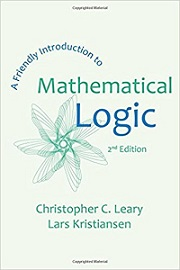Book Description

At the intersection of mathematics, computer science, and philosophy, mathematical logic examines the power and limitations of formal mathematical thinking. In this expansion of Leary's user-friendly 1st edition, readers with no previous study in the field are introduced to the basics of model theory, proof theory, and computability theory.

The text is designed to be used either in an upper division undergraduate classroom, or for self study. Updating the 1st Edition's treatment of languages, structures, and deductions, leading to rigorous proofs of Gödel's First and Second Incompleteness Theorems, the expanded 2nd Edition includes a new introduction to incompleteness through computability as well as solutions to selected exercises.

• Christopher C. Leary, from Homewood, Illinois, was educated at Oberlin College and the University of Michigan, where he wrote his dissertation under the direction of Andreas Blass in 1985. He has held teaching positions at Oberlin, Stetson University, and at SUNY Geneseo, where he has been since 1992. A recipient of the SUNY Chancellor's Award for Excellence in Teaching and the MAA Seaway Section's Clarence F. Stephens Distinguished Teaching Award, he has published work in set theory and in mathematical biology.
• Lars Kristiansen is a professor of Informatics and Mathematics at University of Oslo.
Reviews, Ratings, and Recommendations: Related Book Categories: Read and Download Links:Similar Books:
•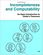Incompleteness and Computability: Gödel's Theorems

This book is an introduction to metamathematics and Gödel's Theorems. It covers recursive function theory, arithmetization of syntax, the first and second incompleteness theorem, models of arithmetic, second-order logic, and the lambda calculus.

•Introduction to Mathematical Logic (Vilnis Detlovs, et al)

This book explores the principal topics of mathematical logic. It covers propositional logic, first-order logic, first-order number theory, axiomatic set theory, and the theory of computability. Discusses the major results of Gödel, Church, Kleene, Rosser, and Turing.

•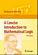A Concise Introduction to Mathematical Logic (W. Rautenberg)

This is a well-written introduction to the beautiful and coherent subject of mathematical logic. It contains classical material such as logical calculi, beginnings of model theory, and Goedel's incompleteness theorems, as well as some topics motivated by applications.

•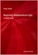Beginning Mathematical Logic: A Study Guide (Peter Smith)

It introduces the core topics and recommends the best books for studying these topics enjoyably and effectively. An invaluable resource both for those wanting to teach themselves new areas of logic and for those looking for supplementary reading.

•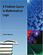A Problem Course in Mathematical Logic (Stefan Bilaniuk)

It is intended to serve as the text for an introduction to mathematical logic for undergraduates with some mathematical sophistication. It supplies definitions, statements of results, and problems, along with some explanations, examples, and hints.

•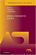Model-Theoretic Logics (Jon Barwise, et al)

This book brings together several directions of work in model theory between the late 1950s and early 1980s. It provides an introduction to the subject as a whole, as well as to the basic theory and examples. Many chapters can be read independently.

•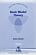Basic Model Theory (Kees Doets)

As the title indicates, this book introduces the reader to what is basic in model theory. A special feature is its use of the Ehrenfeucht game by which the reader is familiarised with the world of models.

•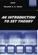An Introduction to Set Theory (William A. R. Weiss)

This book covers the basics: relations, functions, orderings, finite, countable, and uncountable sets, and cardinal and ordinal numbers, gives students sufficient grounding in a rigorous approach to the revolutionary results of set theory.

•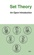Set Theory: An Open Introduction (Richard Zach)

This book is a brief introduction to the philosophy of set theory. It is written for students with a little background in logic, and some high school mathematics, aims to scratch the tip of the surface of the philosophy of set theory.

•Language, Proof and Logic (Jon Barwise, et al)

This book covers first-order language in a method appropriate for first and second courses in logic, and is specially useful to undergraduates of philosophy, computer science, mathematics, and linguistics.

Book Categories
 :All CategoriesTop Free BooksRecent BooksMiscellaneous BooksComputer EngineeringComputer LanguagesComputer ScienceData Science/DatabasesJava and Java EE (J2EE)Linux and UnixMathematicsMicrosoft and .NETMobile ComputingNetworking and CommunicationsSoftware EngineeringSpecial TopicsWeb Programming
Other Categories